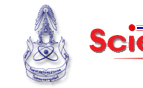| Home  | About ScienceAsia  | Publication charge  | Advertise with us  | Subscription for printed version  | Contact us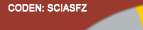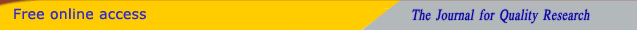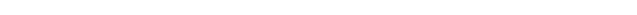Editorial Board Journal Policy Instructions for Authors Online submission Author Login Reviewer Login Volume 49 Number 4 Volume 49 Number 3 Volume 49 Number 2 Volume 49 Number 1 Volume 48 Number 6 Volume 48 Number 5 Earlier issuesVolume 34 Number 4Volume 35 Number 1 Volume 35 Number 2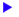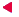previous article next articleResearch articles

ScienceAsia 35 (2009): 70-79 |doi: 10.2306/scienceasia1513-1874.2009.35.070

# A deterministic spectral method for solving the Boltzmann equation for one-dimensional flows

## Chatchawan Watchararuangwita,*, Yurii N. Grigorievb, Sergey V. Meleshkoa

ABSTRACT:     A new deterministic numerical method for solving the kinetic Boltzmann equation for Maxwellian molecules with cylindrical symmetry in velocity space is developed. Using the splitting method with respect to physical processes, the Boltzmann equation is decomposed into the space-homogeneous Boltzmann equation and the transport equation. The transport equation is solved by either Lax-Wendroff or upwind schemes. For Maxwell's model, the space-homogeneous Boltzmann equation is simplified by taking the Fourier transform with respect to velocity. Because of the cylindrical symmetry in velocity space, the three-dimensional Fourier transform is equivalent to a one-dimensional Fourier transform and a Hankel transform. An exponential grid in velocity space allows the application a fast Fourier transform algorithm to compute the Hankel transform. The space homogeneous Boltzmann equation in Fourier space is solved by the Runge-Kutta scheme. The new method is applied to solving the heat transfer problem between parallel plates.